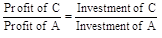# Sample Aptitude Questions of Ittiam

1. What will be the compound interest on Rs. 18,600/- for 2 years, the rate of interest for first year being 8% and for the second year being 15%?
1. Rs. 4489.90
2. Rs. 4967.20
3. Rs. 4232.80
4. Rs. 4501.20
5. Rs. 3637.10

= 18600(1+8/100)(1+15/100)
⇒18600 (1.08) (1.15) = 23101.2
⇒CI = A – P = 23101.2 – 18600 = 4501.2
Hence, Option 4.
2. The sum of five numbers is 260. The average of the first two numbers is 30 and the average of the last two numbers is 70. What is the third numbers?
1. 33
2. 75
3. 60
4. Cannot be determined
5. None of these

a+b+c+d+e=260
⇒a+b /2 =30
⇒ a+b=60
⇒ d+e /2 =70
⇒ d + e =140
⇒ 60+c+140=260
⇒ c = 60
Hence , Option 3.
1. A certain number of capsules were purchased for Rs.176/-. 6 more capsules could have been purchased in the same amount if each capsule was cheaper by Rs. 3/-.What was the number of capsules purchased?
1. 13
2. 16
3. 17
4. 8
5. 11

Let ‘n’ be the number of capsules purchased and ‘c’ be the cost of each capsule. cn = 176
(n+ 6) (c – 3) = 176
Now check option
When n = 16, c = 11
Hence, Option 2.
2. Ram was asked to find 7/8th of a fraction but made the error dividing the fraction by 7/8. As a result of this, he was off the correct answer by 75/784. What answer was Ram supposed to arrive at?
1. 13/32
2. 9/144
3. 5/16
4. 5/14
5. 9/16

ATQ (8/7)y - (7/8)y = 75/784 where y is the req. fraction.
y= 5/14.
Ram is supposed to arrive at (7/8)y = (7/8)*(5/14) = 5/16.
Hence, Option 3.
3. 36 workers can finish a piece of work in 14 days. If the work is to be completed in 8 days, how many extra workers are required
1. 29
2. 33
3. 23
4. 31
5. 27

Let ‘x’ be the extra workers required
 work workers Days w 36 14 w 36+x 8
⇒ 36*14 = (36 + x).8
⇒ on solving x = 27
Hence, Option 5.
4. A man can row 13 kmph downstream and 9 kmph upstream. What is the speed of the man in still water? (in kmph)
1. 12
2. 10.5
3. 11
4. 10
5. 11.5

Speed of stream = s
Speed of man in still water = b
ATQ
b + s = 13
b – s = 9
⇒ b = 11, s = 2
Hence, Option 3.
5. A is 60% more efficient than B. In how many days will ‘A’ and ‘B’ working together complete a piece of work which ‘A’ alone takes 15 days to finish?
1. 124/13
2. 118/3
3. 56/3
4. 131/3
5. 120/13

As A is 60% more efficient than B
⇒ We can suppose that if B does 5 units/days then A does 8 units/day.
Work done by A in 15 days = 120 units.
A and B working together do 13 units in a day.
⇒ A and B can do 120 units of work in
120/13 Days.
Hence, Option 5.
6. The sum of the digits of a two-digit number is 12 and when the digits of the two-digit number are interchanged, the new number is 36 more than the original number. What is the original two digit number?
1. Cannot be determined
2. 93
3. 48
4. 39
5. 84

Let ‘ab’ be the Required Number
a+b=12….(i)
ATQ ba=ab+36
10b+a=10a+b+36
9(b-a)=36
b-a=4….(ii)
From eqn. (i) and (ii)
b=8, a=4
⇒ Required Number = 48, Hence, Option (3).
7. A car covers the first 39 kms of its Journey in 40 minutes & covers the remaining 25 kms in 35 minutes. What is the average speed of the car?
1. 40 km/hr
2. 164 km/hr
3. 49 km/hr
4. 48 km/hr
5. None of these

Average Speed = Total Distance Traveled / Total Time Taken
= (64*60)/75 = 51.2 km/hr.
Hence,Option 5.
8. A, B and C entered into a partnership by investing Rs. 64,000/-, Rs. 52,000/- and Rs. 36,000/- respectively. All of them invested for equal period of time. If A got Rs. 35,584/- as his share of annual profit, what amount did C get as his share of annual profit?
1. Rs. 20,632/-
2. Rs. 18,296/-
3. Rs. 21,084/-
4. Rs. 19,768/-
5. Rs. 20,016/-